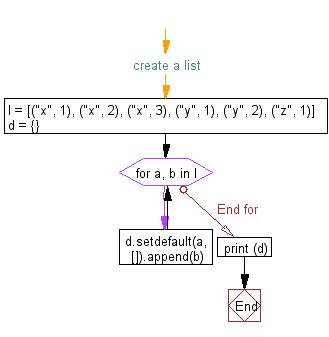﻿ Python: Converting a list of tuples into a dictionary - w3resource# Python Exercise: Converting a list of tuples into a dictionary

## Python tuple: Exercise-19 with Solution

Write a Python program to convert a list of tuples into a dictionary.

Sample Solution:-

Python Code:

``````#create a list
l = [("x", 1), ("x", 2), ("x", 3), ("y", 1), ("y", 2), ("z", 1)]
d = {}
for a, b in l:
d.setdefault(a, []).append(b)
print (d)
```
```

Sample Output:

```{'x': [1, 2, 3], 'y': [1, 2], 'z': }
```

Flowchart:## Visualize Python code execution:

The following tool visualize what the computer is doing step-by-step as it executes the said program:

Python Code Editor:

Have another way to solve this solution? Contribute your code (and comments) through Disqus.

What is the difficulty level of this exercise?

Test your Programming skills with w3resource's quiz.

﻿

## Python: Tips of the Day

Decapitalizes the first letter of a string:

Example:

```def tips_decapitalize(s, upper_rest=False):
return s[:1].lower() + (s[1:].upper() if upper_rest else s[1:])
print(tips_decapitalize('PythonTips'))
print(tips_decapitalize('PythonTips', True))
```

Output:

```pythonTips
pYTHONTIPS
```